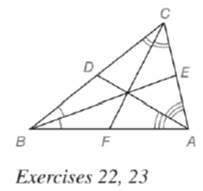Chapter 5.6, Problem 23EElementary Geometry For College St...

7th Edition
Alexander + 2 others
ISBN: 9781337614085

Solutions

Chapter
SectionElementary Geometry For College St...

7th Edition
Alexander + 2 others
ISBN: 9781337614085
Textbook Problem

In Δ A B C , A C = 5.3 , B C = 7.2 and B A = 6.7. With angle bisectors as shown, which line segment is longer?a) A E ¯ or E C ¯ ? b) C D ¯ or D B ¯ ? c) A F ¯ or F B ¯ ?

To determine

a)

To find:

Which line segment is longer

AE¯ or EC¯?

Explanation

Angle-Bisector Theorem:

If a ray bisects one angle of a triangle, then it divides the opposite side into segments whose lengths are proportional to the lengths of the two sides that form the bisected angle.

Calculation:

Given,

AC=5.3,BC=7.2andBA=6.7

From Angle-Bisector theorem,

In ΔABC

ECBC=EABA.........(1)

Let EC=x,

EA=CACE......(2)

Substitute EC=x and AC=5.3 in equation (2) we get,

EA=5.3x

Substitute EA=5.3x,BC=7.2andBA=6

To determine

b)

To find:

Which line segment is longer

CD¯ or DB¯

To determine

c)

To find:

Which line segment is longer

AF¯ or FB¯

Still sussing out bartleby?

Check out a sample textbook solution.

See a sample solution

The Solution to Your Study Problems

Bartleby provides explanations to thousands of textbook problems written by our experts, many with advanced degrees!

Get Started

What is the defining characteristic of a repeated-measures or within-subjects research design?

Essentials of Statistics for The Behavioral Sciences (MindTap Course List)

In Exercises 5-8, graph the given function or equation. y=2x+5

Finite Mathematics and Applied Calculus (MindTap Course List)

limx6(x210x)=. a) 24 b) 6 c) 26 d) does not exist

Study Guide for Stewart's Single Variable Calculus: Early Transcendentals, 8th